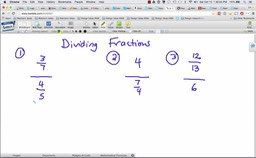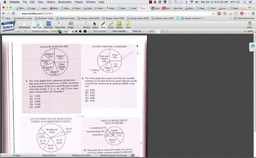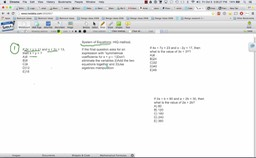## Watch Sample Lessons—Math

Lesson 1: (Beginner's) Dividing Fractions

Lesson 2: (Intermediate) HIQ (High IQ) Method
to Solve "Pie Graph and Percent” Problems

Lesson 3: (Advanced) HIQ Method to Solve a System of Equations Problem, like:

If 4x + 7y = 23 and
x - 2y   = 17, then what is the value of 3x + 3y?
a)8
b)24     c) 32    d) 40   e)48### Lesson 1 (Beginner's) Dividing Fractions AZ / MN### Lesson 2 (Intermediate) Pie Graphs and Percents HIQ